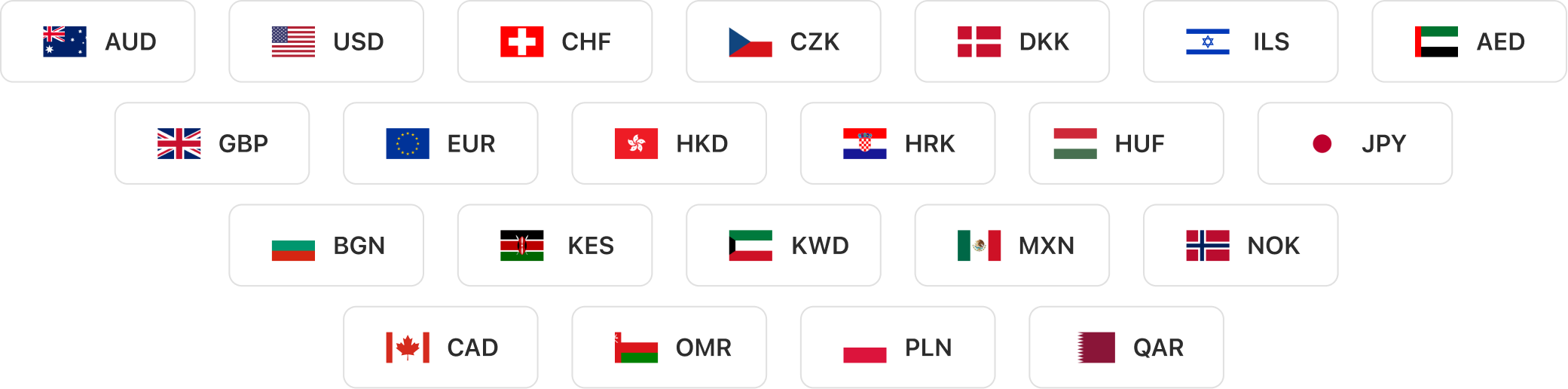# Multi-Currency Account for Remittanceself.__wrap_b=(t,n,e)=>{e=e||document.querySelector(`[data-br="\${t}"]`);let s=e.parentElement,r=R=>e.style.maxWidth=R+"px";e.style.maxWidth="";let o=s.clientWidth,i=s.clientHeight,c=o/2-.25,l=o+.5,u;if(o){for(;c+1<l;)u=Math.round((c+l)/2),r(u),s.clientHeight===i?l=u:c=u;r(l*n+o*(1-n))}e.__wrap_o||(e.__wrap_o=new ResizeObserver(()=>{self.__wrap_b(0,+e.dataset.brr,e)})).observe(s)};self.__wrap_b(":R19db6:",1)

Open multi-currency accounts in seconds. Send and receive currencies anywhere, anytime.## Connected to banks in over 180 countriesself.__wrap_b=(t,n,e)=>{e=e||document.querySelector(`[data-br="\${t}"]`);let s=e.parentElement,r=R=>e.style.maxWidth=R+"px";e.style.maxWidth="";let o=s.clientWidth,i=s.clientHeight,c=o/2-.25,l=o+.5,u;if(o){for(;c+1<l;)u=Math.round((c+l)/2),r(u),s.clientHeight===i?l=u:c=u;r(l*n+o*(1-n))}e.__wrap_o||(e.__wrap_o=new ResizeObserver(()=>{self.__wrap_b(0,+e.dataset.brr,e)})).observe(s)};self.__wrap_b(":R1adb6:",1)

Send and receive remittance from over 180 countries in the chosen currency## Convert Currencies in the Bano Appself.__wrap_b=(t,n,e)=>{e=e||document.querySelector(`[data-br="\${t}"]`);let s=e.parentElement,r=R=>e.style.maxWidth=R+"px";e.style.maxWidth="";let o=s.clientWidth,i=s.clientHeight,c=o/2-.25,l=o+.5,u;if(o){for(;c+1<l;)u=Math.round((c+l)/2),r(u),s.clientHeight===i?l=u:c=u;r(l*n+o*(1-n))}e.__wrap_o||(e.__wrap_o=new ResizeObserver(()=>{self.__wrap_b(0,+e.dataset.brr,e)})).observe(s)};self.__wrap_b(":R1bdb6:",1)

Use Bano FX Converter to exchange currencies at the best rates with no hidden transaction fees!## Support of up to 37 currenciesself.__wrap_b=(t,n,e)=>{e=e||document.querySelector(`[data-br="\${t}"]`);let s=e.parentElement,r=R=>e.style.maxWidth=R+"px";e.style.maxWidth="";let o=s.clientWidth,i=s.clientHeight,c=o/2-.25,l=o+.5,u;if(o){for(;c+1<l;)u=Math.round((c+l)/2),r(u),s.clientHeight===i?l=u:c=u;r(l*n+o*(1-n))}e.__wrap_o||(e.__wrap_o=new ResizeObserver(()=>{self.__wrap_b(0,+e.dataset.brr,e)})).observe(s)};self.__wrap_b(":Rcdb6:",1)

More currencies to come soon